Get Free NCERT Solutions for Class 10 Maths Chapter 3 Ex 3.2 PDF. Pair of Linear Equations in Two Variables Class 10 Maths NCERT Solutions are extremely helpful while doing your homework. Exercise 3.2 Class 10 Maths NCERT Solutions were prepared by Experienced LearnCBSE.in Teachers. Detailed answers of all the questions in Chapter 3 Maths Class 10 Pair of Linear Equations in Two Variables Exercise 3.2 provided in NCERT TextBook.

Topics and Sub Topics in Class 10 Maths Chapter 3 Pair of Linear Equations in Two Variables:

 Section Name Topic Name 3 Pair of Linear Equations in Two Variables 3.1 Introduction 3.2 Pair Of Linear Equations In Two Variables 3.3 Graphical Method Of Solution Of A Pair Of Linear Equations 3.4 Algebraic Methods Of Solving A Pair Of Linear Equations 3.4.1 Substitution Method 3.4.2 Elimination Method 3.4.3 Cross-Multiplication Method 3.5 Equations Reducible To A Pair Of Linear Equations In Two Variables 3.6 Summary

You can also download the free PDF of  Ex 3.2 Class 10 Pair of Linear Equations in Two Variables NCERT Solutions or save the solution images and take the print out to keep it handy for your exam preparation.

 Board CBSE Textbook NCERT Class Class 10 Subject Maths Chapter Chapter 3 Chapter Name Pair of Linear Equations in Two Variables Exercise Ex 3.2 Number of Questions Solved 7 Category NCERT Solutions

## NCERT Solutions for Class 10 Maths Chapter 3 Pair of Linear Equations in Two Variables Ex 3.2

NCERT Solutions for Class 10 Maths Chapter 3 Pair of Linear Equations in Two Variables Ex 3.2 are part of NCERT Solutions for Class 10 Maths. Here we have given NCERT Solutions for Class 10 Maths Chapter 3 Pair of Linear Equations in Two Variables Exercise 3.2

Ex 3.2 Class 10 Maths Question 1.
Form the pair of linear equations of the following problems and find their solutions graphically:
(i) 10 students of class X took part in a Mathematics quiz. If the number of girls is 4 more than the number of boys, find the number of boys and girls who took part in the quiz.
(ii) 5 pencils and 7 pens together cost ₹50, whereas 7 pencils and 5 pens together cost ₹46. Find the cost of one pencil and that of one pen.
Solution: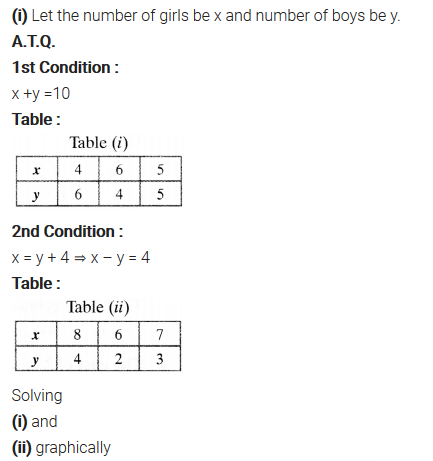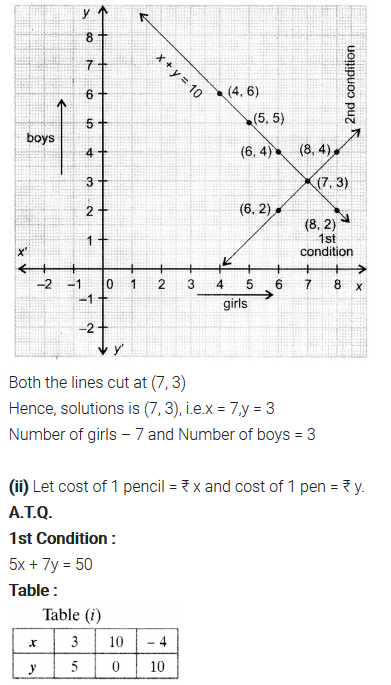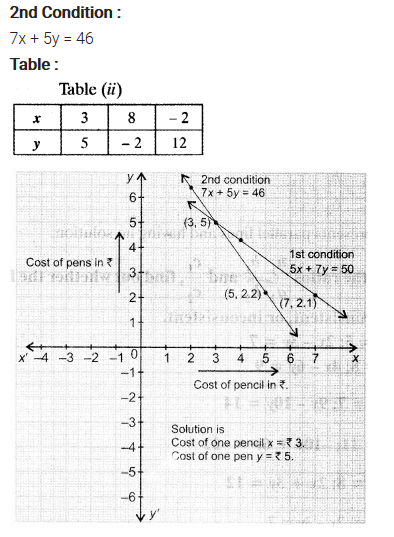Ex 3.2 Class 10 Maths Question 2.
On comparing the ratios $$\frac { { a }_{ 1 } }{ { a }_{ 2 } }$$, $$\frac { { b }_{ 1 } }{ { b }_{ 2 } }$$
and $$\frac { { c }_{ 1 } }{ { c }_{ 2 } }$$ , find out whether the lines representing the following pairs of linear equations intersect at a point, are parallel or coincident:
(i) 5x – 4y + 8 = 0, 7x + 6y – 9 = 0
(ii) 9x + 3y + 12 = 0, 18x + 6y + 24 = 0
(iii) 6x – 3y + 10 = 0, 2x -y + 9 = 0
Solution: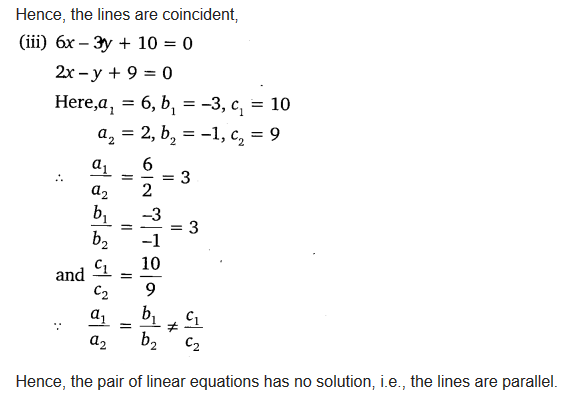Ex 3.2 Class 10 Maths Question 3.
On comparing the ratios $$\frac { { a }_{ 1 } }{ { a }_{ 2 } }$$, $$\frac { { b }_{ 1 } }{ { b }_{ 2 } }$$
and $$\frac { { c }_{ 1 } }{ { c }_{ 2 } }$$, find out whether the following pairs of linear equations are consistent, or inconsistent:
~
Solution: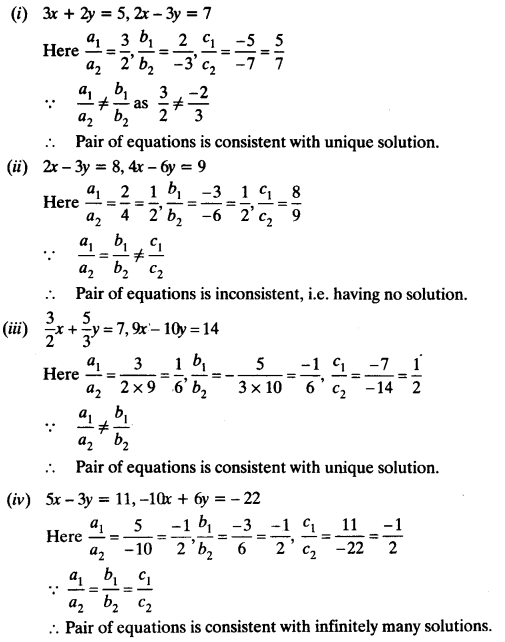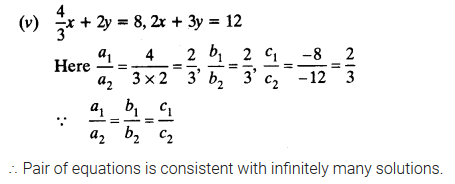Ex 3.2 Class 10 Maths Question 4.
Which of the following pairs of linear equations are consistent/inconsistent? If consistent, obtain the solution graphically.
(i) x + y = 5, 2x + 2y = 10
(ii) x-y – 8, 3x – 3y = 16
(iii) 2x + y – 6 = 0, 4x – 2y – 4 = 0
(iv) 2x – 2y – 2 = 0, 4x – 4y – 5 = 0
Solution: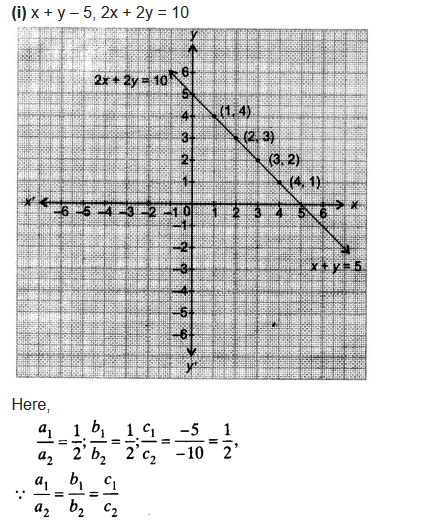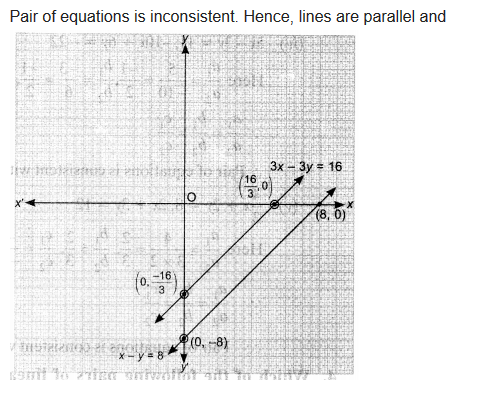Ex 3.2 Class 10 Maths Question 5.
Half the perimeter of a rectangular garden, whose length is 4 m more than its width is 36 m. Find the dimensions of the garden graphically.
Solution: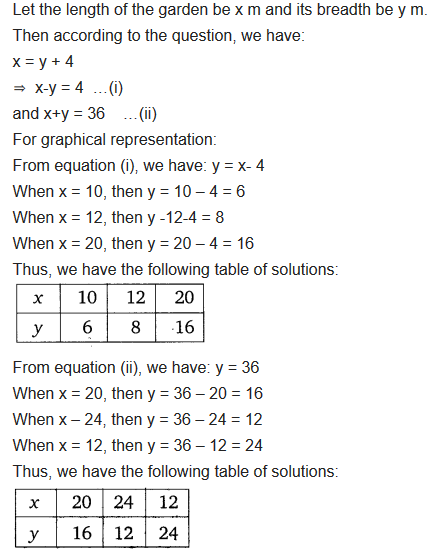Ex 3.2 Class 10 Maths Question 6.
Given the linear equation 2x + 3y – 8 = 0, write another linear equation in two variables such that the geometrical representation of the pair so formed is:
(i) intersecting lines
(ii) parallel lines
(iii) coincident lines
Solution: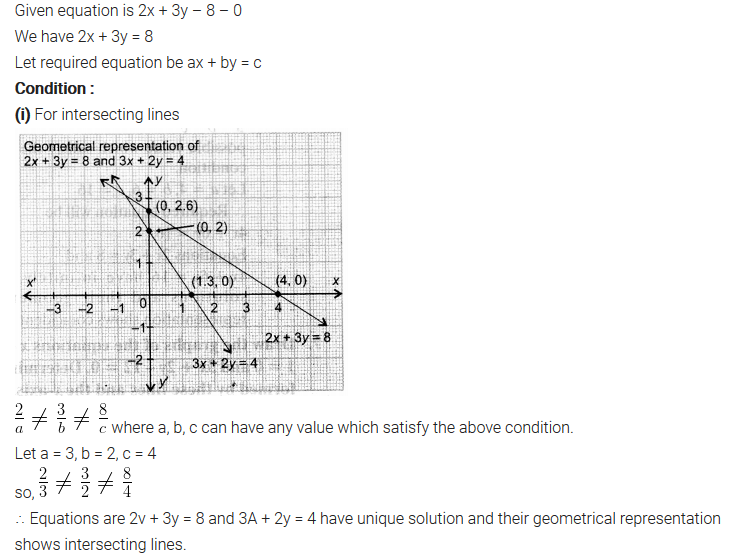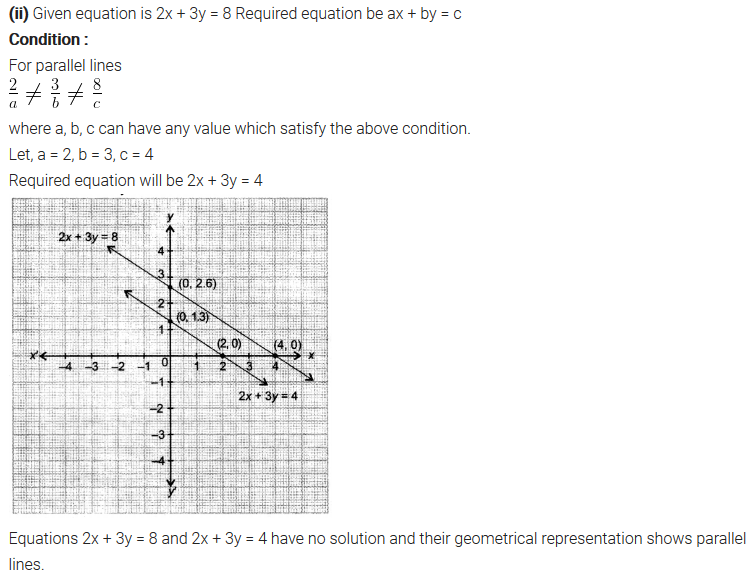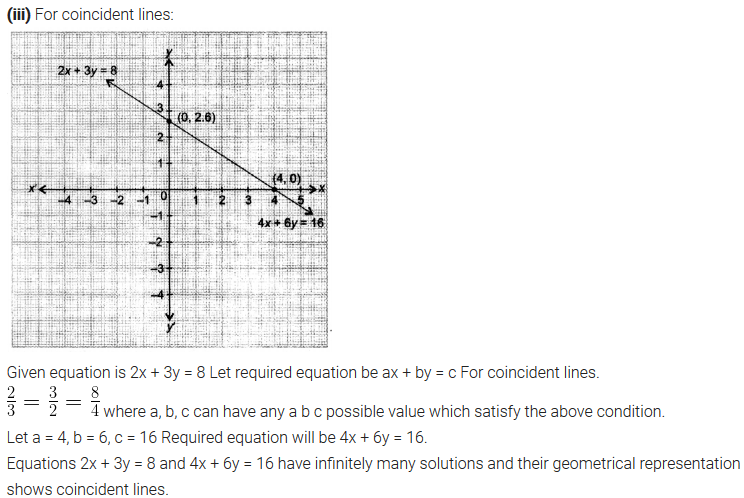Ex 3.2 Class 10 Maths Question 7.
Draw the, graphs of the equations x – y + 1 = 0 and 3x + 2y – 12 = 0. Determine the coordinates of the vertices of the triangle formed by these lines and the x-axis, and shade the triangular region.
Solution:### NCERT Solutions for Class 10 Maths Chapter 3 Pairs of Linear Equations in Two Variables (Hindi Medium) Ex 3.2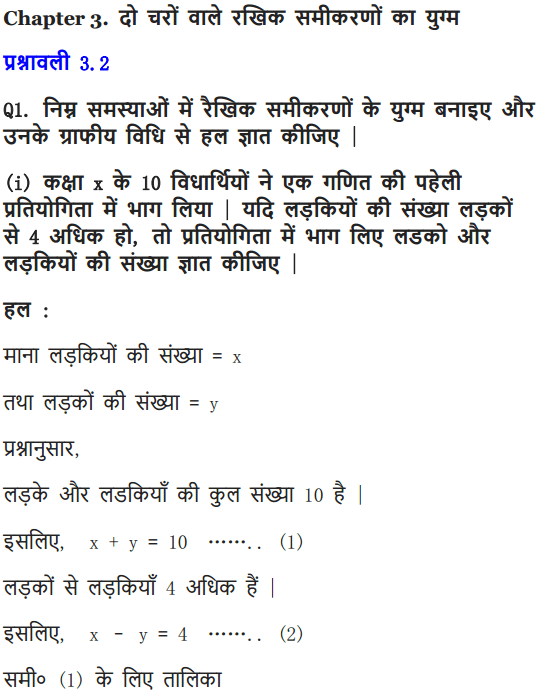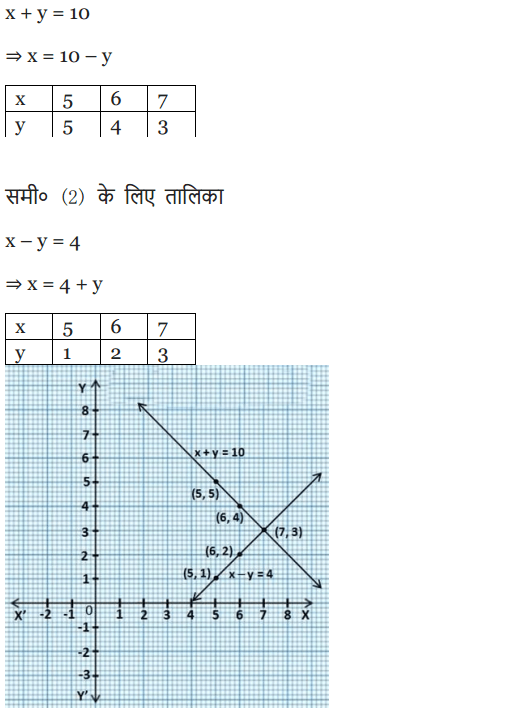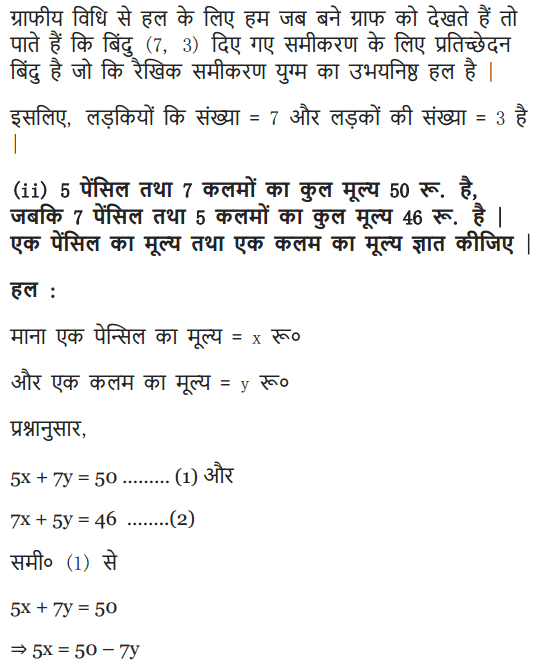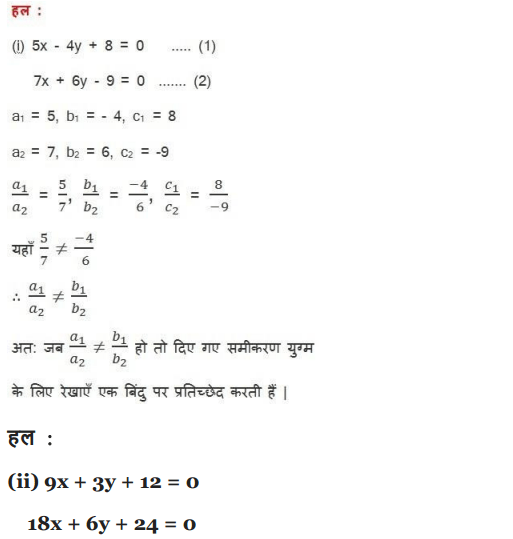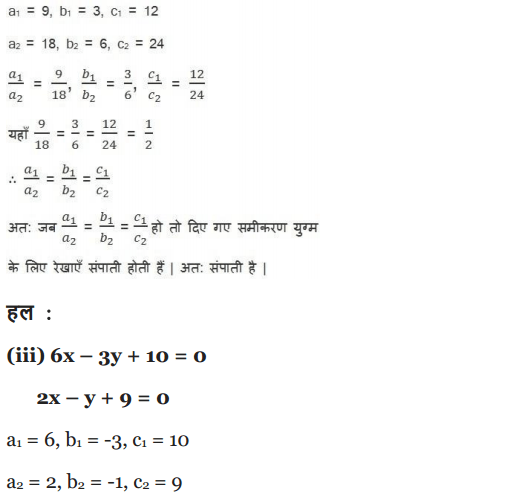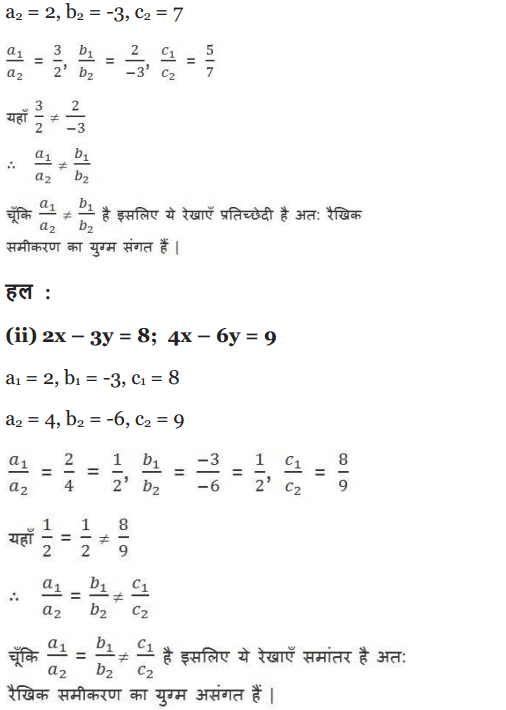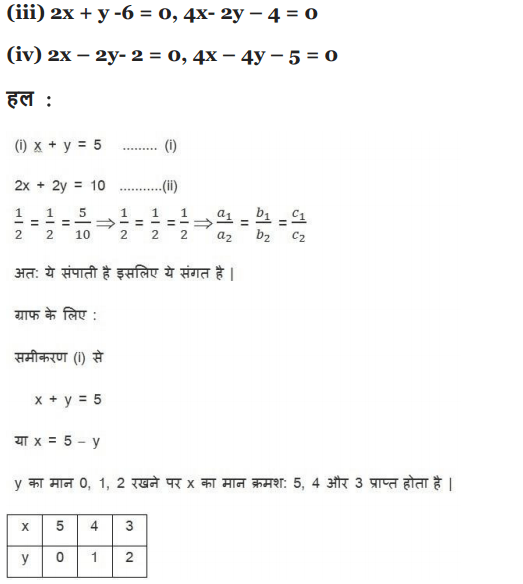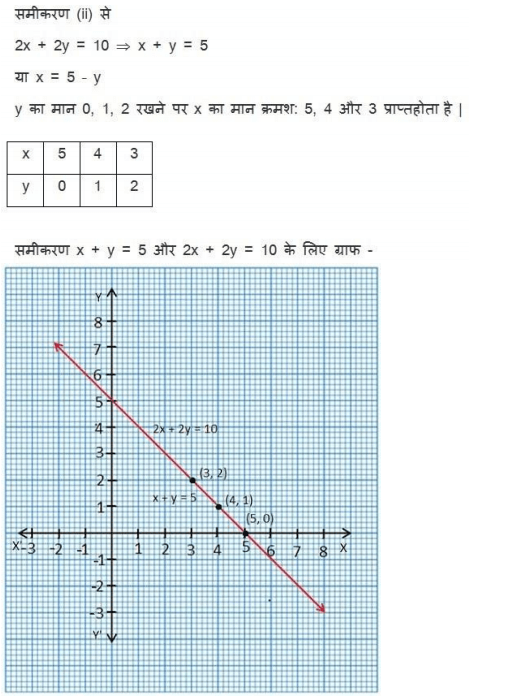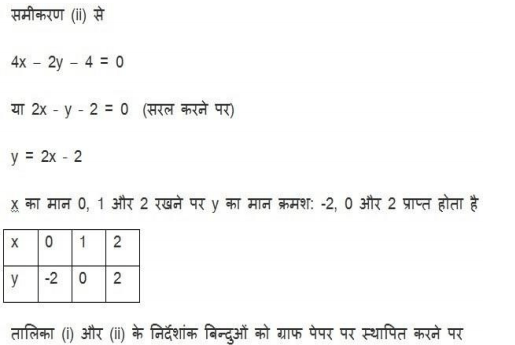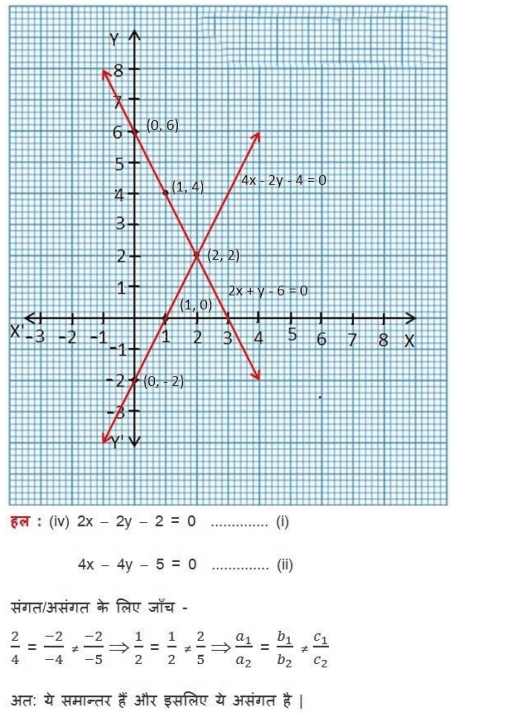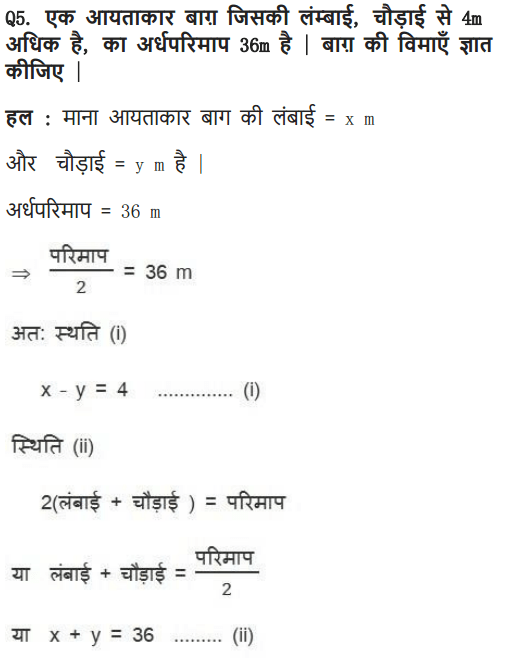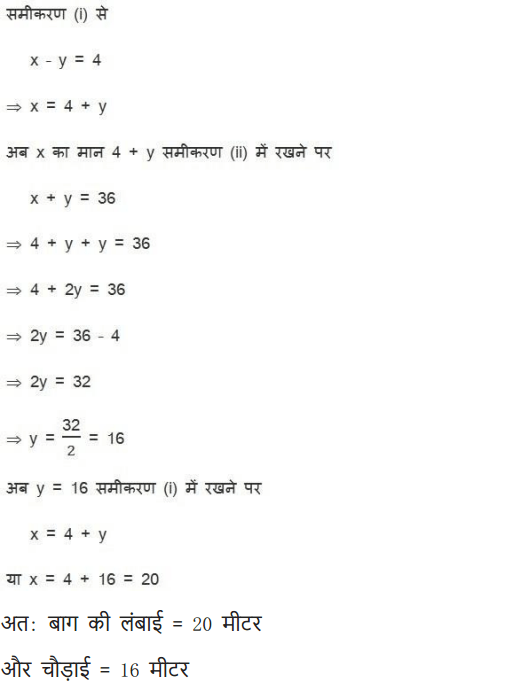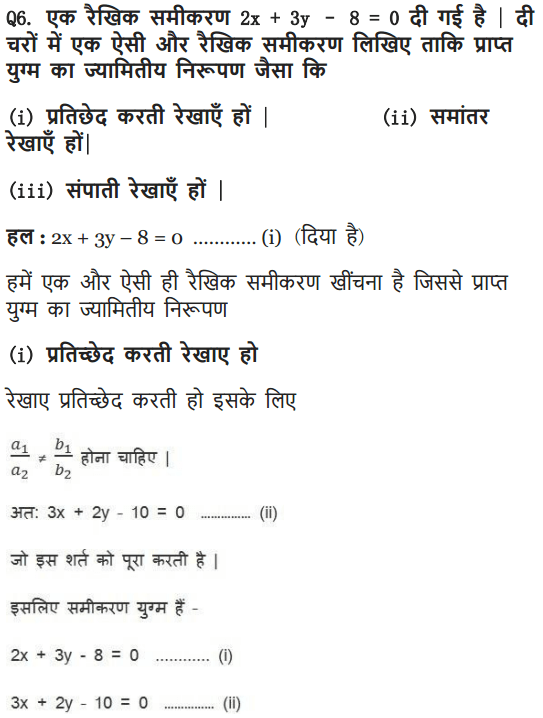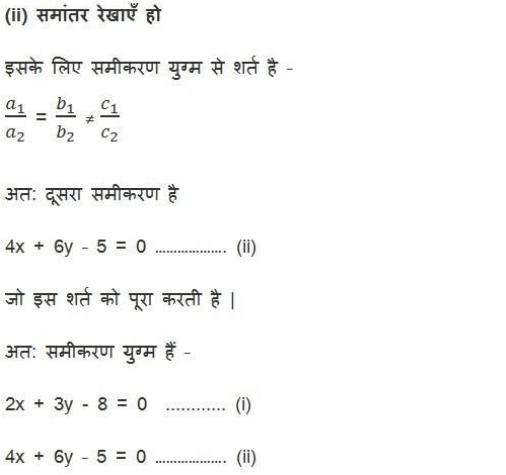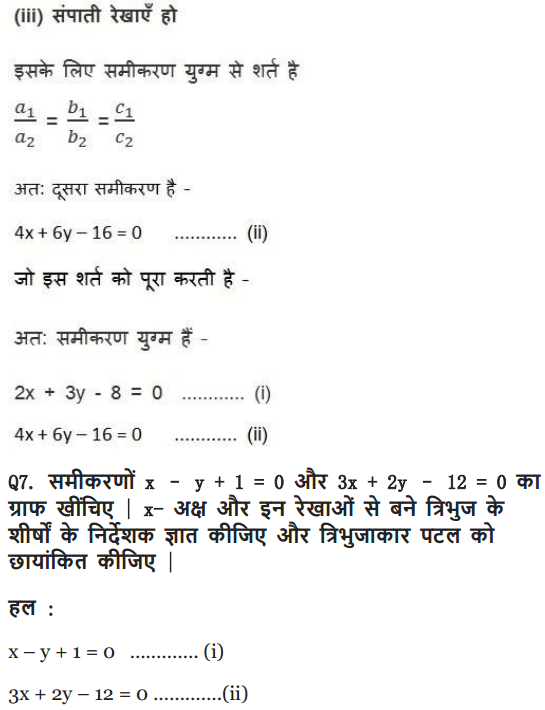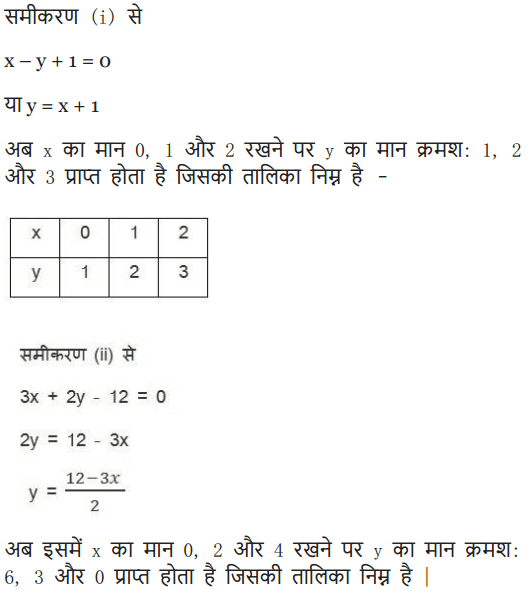We hope the NCERT Solutions for Class 10 Maths Chapter Pair of Linear Equations in Two Variables Ex 3.2, help you. If you have any query regarding NCERT Solutions for Class 10 Maths Chapter 3 Pair of Linear Equations in Two Variables Exercise 3.2, drop a comment below and we will get back to you at the earliest.